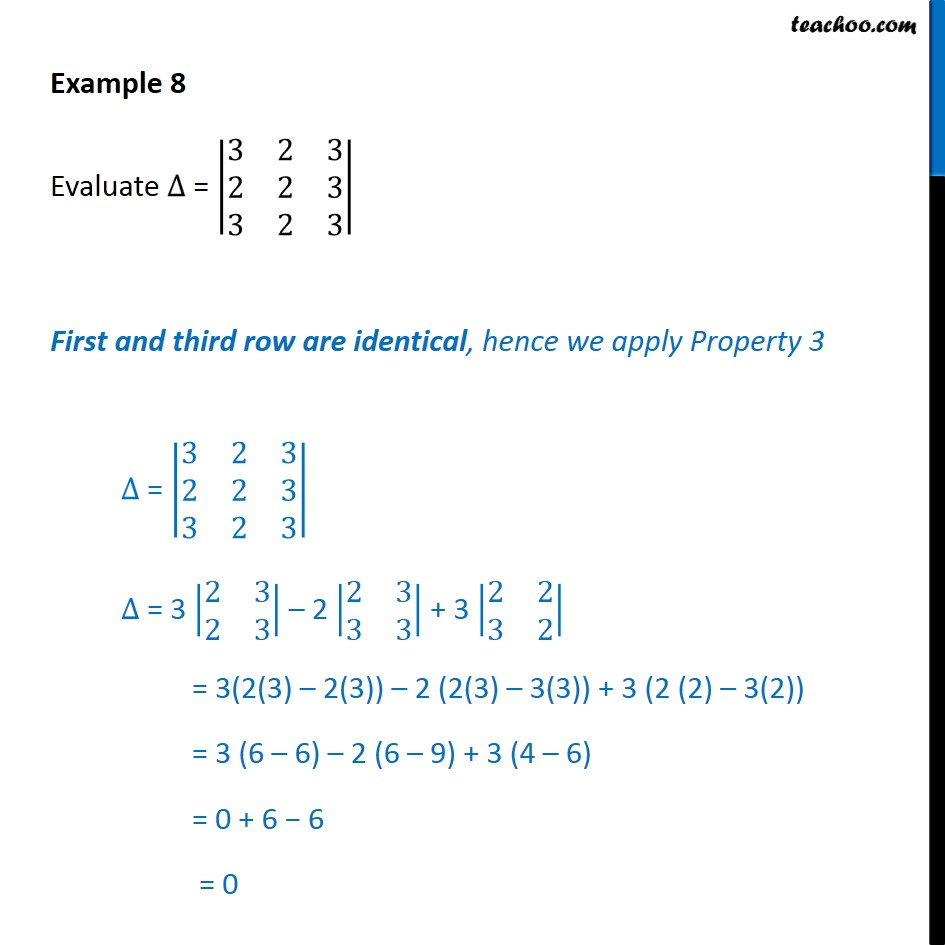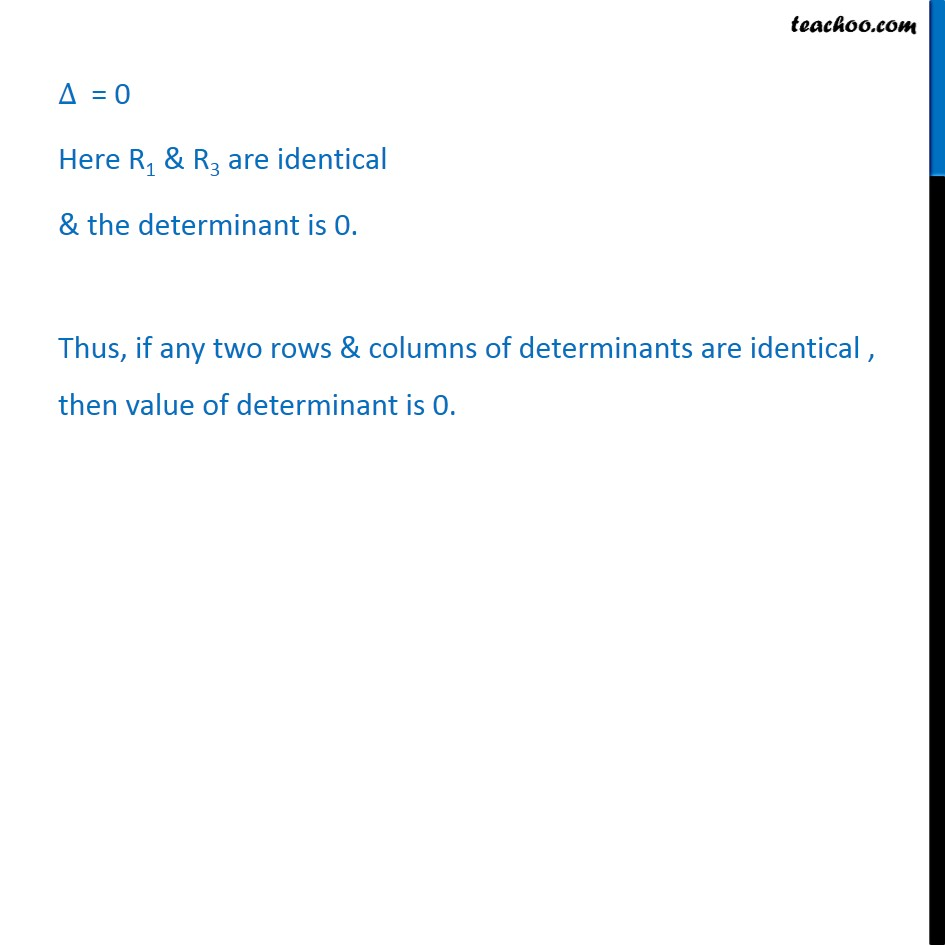1. Chapter 4 Class 12 Determinants (Term 1)
2. Serial order wise
3. Examples

Transcript

Example 8 Evaluate ∆ = 3﷮2﷮3﷮2﷮2﷮3﷮3﷮2﷮3﷯﷯ First and third row are identical, hence we apply Property 3 ∆ = 3﷮2﷮3﷮2﷮2﷮3﷮3﷮2﷮3﷯﷯ ∆ = 3 2﷮3﷮2﷮3﷯﷯ – 2 2﷮3﷮3﷮3﷯﷯ + 3 2﷮2﷮3﷮2﷯﷯ = 3(2(3) – 2(3)) – 2 (2(3) – 3(3)) + 3 (2 (2) – 3(2)) = 3 (6 – 6) – 2 (6 – 9) + 3 (4 – 6) = 0 + 6 − 6 = 0 ∆ = 0 Here R1 & R3 are identical & the determinant is 0. Thus, if any two rows & columns of determinants are identical , then value of determinant is 0.